RUSSIAN JOURNAL OF EARTH SCIENCES, VOL. 19, ES1005, doi:10.2205/2019ES000662, 2019

# Estimation of the wind-driven wave spectrum using a high spatial resolution coherent radar

A. V. Ermoshkin, I. A. Kapustin

Institute of Applied Physics of the Russian Academy of Sciences, Nizhniy Novgorod, Russia

### Abstract

The paper deals with experimental determination of the wind-driven wave spectrum by using the remote method of high-resolution coherent radar sensing of the water surface. The method is applied to the conditions of the fetch-limited wind wave growth, which is typical for enclosed waters and the sea nearshore, where the dominant wavelength is of the order of ten meters. The experiments were performed using a coherent X-band panoramic digital radar operating with the horizontal polarization for transmission and reception. The paper considers an algorithm used to form velocity images of the water surface from the data of coherent radar sensing on the basis of the phase angle difference method. The paper shows the possibility to recover wind wave spectra from the data on the Doppler shift of microwave radio waves. A theoretical justification and an experimental verification of the method are provided. Appearance of the third harmonic of the wind waves recovered from the radar sensing data of the spatio-temporal spectra of Doppler velocities is demonstrated for the first time. The functions that relate to the wave elevation spectra and the Doppler velocity spectra are determined. It is shown that the linear free-surface wave approximation is valid for the reconstruction of wave spectra. The temporal and spatial omnidirectional wave spectra obtained in the experiment are described well by the power model functions using the exponent factor of $-4$ and $-5/2$, respectively. The presented results may be used for studies of interaction between the harmonics of wind waves with the bottom topography, wave-current interaction, diagnostic of non-homogeneous currents, and other hydrophysical processes.

### Introduction

The purpose of this work is to study the possibility of remote radar measurement of spatio-temporal spectra of wind waves for the meter and decameter ranges. In well-known papers [Lyzenga et al., 2010] that used the data from pulse radar, the spatial resolution was 10 m, while in [Plant and Farquharson, 2012], it was 7.5 m. Thus, the spatial spectra of radar images were limited to a wave number of 0.3–0.4 rad/m. This is not sufficient for analysis of the wave spectra in the fetch-limited conditions, e.g., in the coastal zone and in enclosed water bodies. A significant extension in the range of the measured wavelengths became possible due to a high spatial resolution of the radar used (less than 1 m) and coherence of the radiation. In this work, field experiments were performed on a Stationary Oceanographic Platform (SOP) of the Black Sea Hydrophysical Test Site of the Russian Academy of Sciences in the coastal area of the Black Sea near Katsiveli. During the experiments, velocity radar images of wavy water surface were obtained and used for spectrum processing. Comparative analysis of the temporal and spatial spectra of wind waves, which were determined based on the data obtained with high spatial resolution coherent radar and a contact wave gauge (string wave recorder), was carried out. It has been shown that the wave spectra reconstructed from velocity radar images correlate with the data of roughness contact measurements, both qualitatively and quantitatively.

#### Theoretical Background

Let us consider the problem of propagation of linear free-surface waves and obtain an expression that relates the spectra of the measured Doppler velocities to the spectra of the wave height

[Nekrasov and Pelinovskiy (eds.), 1992]. For linear deep-water waves propagating along the $x$ axis ($kh \gg 1$), the potential may be expressed as

\begin{eqnarray*} \varphi (x,t) = \frac{g}{\omega} \eta_0 \sin (\omega t - kx + \alpha) \end{eqnarray*}

where $\eta_0$ is the wave amplitude, and $\omega$ and $k$ are the frequency and wave number, respectively.

Then, the expression for the horizontal component of velocity has the form

\begin{eqnarray*} u (x,t) = \frac{\partial\varphi }{\partial x}= \frac{g k}{\omega} \eta_0 \cos (\omega t - kx + \alpha) \end{eqnarray*}

while that for the wave amplitude is

\begin{eqnarray*} \eta (x,t) = \frac{1 }{g}\frac{\partial\varphi }{\partial t}= \eta_0 \cos (\omega t - kx + \alpha) \end{eqnarray*}

The relation between the wave elevation spectrum and the horizontal velocity spectrum is determined by the following expression:

\begin{equation} \tag*{(1)} S_{\eta} (k,\omega) = \Bigl( \frac{\omega}{g k} \Bigr)^2 S_u (k,\omega) \end{equation}

The horizontal component of velocity at the sea surface may be determined by measuring the Doppler velocity of the reflecting elements, which may be written as

\begin{eqnarray*} u_d(x, t, \vartheta ) = \end{eqnarray*} \begin{eqnarray*} (u^x_{br} (x) + u (x,t) + u_c^x (x)) \cos \vartheta \sin \theta \end{eqnarray*}

where $\vartheta$ is the azimuth angle of sensing with respect to the wave propagation direction, coinciding with the $x$ axis, $u_c^x$ is the component of the current velocity in the direction of the wave propagation, $u_{br}^x$ is the phase velocity of the scattering (Bragg) wave

\begin{eqnarray*} u_{br}^x = \sqrt{ \frac{ g \lambda}{4 \pi \sin \theta} + \frac{2 \pi \sigma}{\rho\lambda} \sin \theta} \end{eqnarray*}

$\lambda$ is the wavelength of the radar, $\theta$ is the incidence angle, $\sigma$ is the surface tension coefficient, and $\rho$ is the water density. The time-averaged Doppler velocity is

\begin{eqnarray*} \overline{u_d (x, \vartheta)}^T = \end{eqnarray*} \begin{eqnarray*} \overline{(u_{br}^x (x) + u(x,t) + u_{c}^x (x))}^T \cos \vartheta \sin \theta \approx \end{eqnarray*} \begin{eqnarray*} (u_{br}^x (x) + u_{c}^x) \cos \vartheta \sin \theta \end{eqnarray*}

because $u_{br}^x$ and $u_{c}^x$ are time-independent values and $\overline{u (x, t)}^T \approx 0$. Then, it is possible to use (1) to relate the spectrum of the measured Doppler velocities and the wave elevation spectrum with the following linear equation:

\begin{equation} \tag*{(2)} S_{\eta} (k,\omega) = \Bigl( \frac{\omega}{g k \cos \vartheta \sin \theta} \Bigr)^2 S_{\tilde{u}_d} (k,\omega) \end{equation}

where

\begin{eqnarray*} \tilde{u}_d (x, t, \vartheta) = u_d (x, t, \vartheta) - \overline{u_d(x, \vartheta)}^T \end{eqnarray*}

#### Experiment and Data Analysis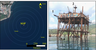Figure 1

To check the theoretical conclusions for the fetch-limited conditions, a field experiment was carried out. A coherent X-band radar system operating with the HH polarization mode was used as a source of the radar data. The radar was installed at a height of 16 m above sea level, and the range of the incident angles $\theta$ was from 40° to 88.2° (Figure 1). The wind velocity, air temperature, pressure, humidity of the near-surface atmospheric layer were measured using a Vantage Pro2 Weather Station (Davis Instruments$^{\mathrm TM}$) installed at a height of 26 m (2 in Figure 1). The marine current velocity at depths from 1 to 25 m with a step of 0.5 m were obtained according to the data of the acoustic Doppler current profiler (ADCP WorkHorse Monitor 1200 kHz, RDI$^{\mathrm TM}$), which was hung out on the cables from the middle deck (3 in Figure 1). The ADCP was oriented vertically down and was sunk to a depth of 0.3 m. The surface elevation was measured with a string wave gauge installed at the SOP (4 in Figure 1).

This coherent radar (Micran$^{\mathrm TM}$) operated in a chirp modulation mode with a modulation bandwidth of $\Delta f = 191$ MHz and an emitted radio power of 1 W. The range resolution was

\begin{eqnarray*} \Delta_r= \frac{c}{2\Delta f} = 0.79 \;{\mathrm m}, \end{eqnarray*}

the azimuth resolution was

\begin{eqnarray*} \Delta_\vartheta= \frac{a \pi R}{360} \end{eqnarray*}

where $a = 1\mbox{°}$ is the angular resolution (the antenna length was 2 m), and $R$ was the distance from the resolution element on the sea surface. Thus, for virtually all conditions of sea disturbance, the condition $\Lambda > 2 \Delta_r$ holds, where $\Lambda$ is the length of the dominant wave, but the condition $\Lambda > 2 \Delta_\vartheta$ holds for $R$ being less than 500 meters. The received beat signal (the difference between the reflected and emitted chirp signals) was subjected to a Fourier transform, which resulted in the vector $Q(r,\vartheta)$ consisting of the amplitude $A$ and phase $\varphi$ of the reflected radar signal at distances of up to 500 m with a step of 0.79 m. Two spatial-scanning modes were used: lateral and circular. When the radar operated in the lateral mode, a sequential set of vectors $Q(r,\vartheta_0, t) = A (r,\vartheta_0, t) e^{i\varphi (r,\vartheta_0, t)}$ was obtained with the time interval $\tau=3.5$ ms between them at a fixed position of the antenna azimuth angle $\vartheta_0$. By fixing the distance $r=r_0$ and applying the Fourier transform to $N$ elements $Q(r_0, \tau:\tau: N\tau, \vartheta_0)$, we may obtain the Doppler spectra $D(f)$ of the reflected radar signal at the distance $r_0$. Numerical simulation of the radar sensing for such a problem statement was given in [Toporkov and Sletten, 2017]. For a rotating antenna, there is a periodic scanning of the environment $Q(r,\vartheta,n_r T_r)$ with the antenna rotation period $T_r$, where $n_r$ is the number of rotations.

Let us consider the algorithm used to determine Doppler velocities based on the data of coherent radar sensing as described in [Pereslegin and Sinitsyn, 2011]. For the $k$th vector, we have the phase value

\begin{eqnarray*} \varphi_k (r, \vartheta_0)= \varphi_0 (r, \vartheta_0) - 2 \pi f_d^\vartheta (r, \vartheta_0) \tau k. \end{eqnarray*}

Then, on the condition of sensing the same area, the difference between the two phases of the neighboring vectors is calculated as

\begin{eqnarray*} \Delta\varphi = \mathrm{arg}(Q(k) \times Q^* (k+1) = \end{eqnarray*} \begin{eqnarray*} \mathrm{arg}\bigl( A^2(r, \vartheta_0) e^{ -i 2 \pi f_d^\vartheta (r, \vartheta_0) \tau} \bigr) = 2 \pi f_d^\vartheta (r, \vartheta_0) \tau. \end{eqnarray*}

Using the ratio

\begin{eqnarray*} f_d^\vartheta= \frac{2 u_d}{\lambda} \sin \theta \end{eqnarray*}

and the equation for the phase difference $\Delta\varphi$, the Doppler velocity may be written as

\begin{eqnarray*} u_d = \frac{\Delta\varphi\lambda}{4 \pi \tau \sin \theta}. \end{eqnarray*}

According to the method described in [Pereslegin and Sinitsyn, 2011], the sensitivity to velocity fluctuations is determined as

\begin{eqnarray*} ( u_d)_{\mathrm{sens}} = \frac{q_0}{\frac{\partial (\Delta\varphi)}{\partial(u_d)} \sqrt{N}}, \end{eqnarray*}

where $q_0 >1$ is the threshold coefficient, $N$ is the number of independent samples of the signal at a given site, and $[\partial (\Delta\varphi)]/[\partial(u_d)]$ is the steepness of the characteristic, which is equal to

\begin{eqnarray*} \frac{\partial (\Delta\varphi)}{\partial(u_d)} = \frac{4 \pi \tau \sin \theta}{\lambda} \approx 1.4 \: \mathrm{rad \;s/m} \end{eqnarray*}

for the parameters of the radar used.

In the lateral scanning mode, brightness- and velocity-based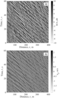Figure 2

$(A(r, \vartheta_0, t)$ and $u_d(r, \vartheta_0, t)$, respectively) radar images of the water surface may be obtained in a distance-time system of coordinates (DTI, distance-time image) (see Figure 2). These images were obtained when scanning upwind in an experiment conducted at a wind velocity of 10 m/s. A wave structure of the image shows a variation in the slope of the stripes relative to the $x$ axis, which is explained by various propagation velocities of the wind-driven wave harmonics.

Statistics of the Doppler velocity distribution $u_d(r, \vartheta_0, t)$ should correspond to the wind wave height distribution function, which is a Gaussian function in the linear approximation under consideration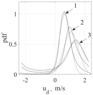Figure 3

[Nekrasov and Pelinovskiy (eds.), 1992]. Figure 3 shows the Doppler velocity distribution functions for various distances (incident angles) shown in Figure 2b. There is an increase in the average value of the distribution function and a broadening with a growing incident angle, which is explained by shadowing of some areas of the water surface with crests of the waves at low grazing angles. However, this trend may be eliminated by subtracting the time average for each distance. The dashed line in Figure 3 shows an approximation of the distribution functions with a Gaussian. For incident angles being not too close to the grazing one (curve 1 in Figure 3), we may conclude that the statistics of distribution of the Doppler velocities is normal.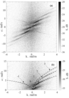Figure 4

Spectral processing of the velocity DTI after subtracting the time-averaged Doppler velocity for each distance gives a spatio-temporal wave spectrum (2) The boundary value of the wave number is determined as $k_N =\pi/\Delta_r \approx 4$ rad/m, which corresponds to the wave frequency in deep water $- \omega(k_N) = \sqrt{g k_N} \approx 6.2 \:\mathrm{rad/s}$. Figure 4 shows the result of the spatio-temporal wave spectrum reconstruction using this approach and employing the data on the Doppler velocities given in Figure 2b.

The areas of energy localization are prominent in the reconstructed wave spectrum. The main share of the energy is concentrated near the dispersion curve $\omega = \sqrt{g k} + k u_c$, where $u_c$ is the velocity of the current at the surface being equal to 0.3 m/s (dashed line 1 in Figure 4b), and the $n$-fold harmonics $nk, \;n\omega$, where $n=2$ (dashed line 2 in Figure 4b) and $n=3$ (dashed line 3 in Figure 4b) [Slunyaev and Sergeeva, 2012]. There are also prominent oncoming waves $\omega = \sqrt{g k} - k u_c$ (dashed line $-1$ in Figure 4b) and the energy area corresponding to the group structure of the wind waves (0 in Figure 4b).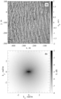Figure 5

The spatio-temporal wave spectrum from the coherent radar data may also be determined in the case of all-round observation of the environment [Trizna, 2011]. The ratio between the antenna rotation period $T_r$ and the pulse repetition period $\tau$ determines $N$, which is a number of the necessary averaged independent measurements of the Doppler velocity in the resolution element on the wavy surface. Under circular scanning conditions, the amplitude $A(r,\vartheta, n_rT_r)$ and velocity $u_d(r,\vartheta, n_rT_r)$ radar images are obtained. As a result of spectral processing of $u_d(r,\vartheta, n_rT_r)$, we get the spatio-temporal wave spectrum $S_\eta (k, \omega,\vartheta)$ with a boundary wave number, $k_N \approx 4$ rad/m, while the limiting frequency for the maximum rotation speed of the antenna is $T_r\approx 2.5$ s, $\omega_N = \pi/T_r \approx 1.2$ rad/s which is significantly smaller than the frequency value for gravity waves on the water surface at $k_N$. This processing, however, is justified for long waves. In [Nwogu and Lyzenga, 2010], the authors reconstruct the elevation of the sea surface from measurements of the Doppler velocities with a rotating antenna in the circular scan mode. In this paper, we consider spectral processing of the velocity image for a single rotation of the antenna. The field of the Doppler velocities $u_d(x,y)$, interpolated to a uniform grid is shown in Figure 5a. The wind speed in this experiment was 12 m/s. The reconstructed two-dimensional wave spectrum determined as

\begin{eqnarray*} S_\eta (k_x, k_y) = \frac{1}{g \sqrt{k_x^2 + k_y^2}} S_{u_d} (k_x, k_y) \end{eqnarray*}

is shown in Figure 5b.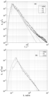Figure 6

The surface elevation was measured with a string wave gauge installed at the SOP (4 in Figure 1), in parallel with the radar observations. The resistive string wave gauge consisted of a three-channel, analogue processing scheme and an analog-to-digital converter connected to a computer. Each channel of the wave gauge included a pair of strings (the string material is cantal A1, string thickness is 0.4 mm) spread by 5 mm, separate channels of the transmitter were spread to the corners of an equilateral triangle with a side of 20 cm. The wave gauge was calibrated by raising and lowering the gauge to a predefined height and plotting the voltage-height transmission curve. The calibration curve was used for recalculation of the measured voltages to wave heights with subsequent plotting of the wind wave spectra. The quantitative comparison between the height spectra of the waves obtained by the contact method and those obtained remotely is given in Figure 6. The frequency spectrum of wind waves was determined based on the coherent radar data as the average in the Doppler velocities ensemble for the angles being not too close to the grazing one and corresponding to curve 1 in Figure 3:

\begin{eqnarray*} S_\eta (\omega) = \frac{1}{N} \sum_{i=1}^{N} \Bigl( \frac{1}{\omega \cos \vartheta_0 \sin \theta_i} \Bigr)^2 S_{\tilde{u}_d} (\omega,\theta_i) \end{eqnarray*}

(solid line in Figure 6a). In the same figure, there is also a height spectrum of the wind wave obtained basing on the wave gauge data (dotted line) and the JONSWAP model spectra with $f^{-5}$ (dot-dash line) and $f^{-4}$ (points) power dependences from paper [Battjes et al., 1987]. There is a nice agreement of the remotely measured spectra with the JONSWAP model spectrum $f^{-4}$. A comparison of the omnidirectional wave spectrum obtained from the spectrum of Doppler velocities is shown in Figure 6b. Then, the wave spectrum recovered from the coherent radar data (solid line in Figure 6b) will be determined as

\begin{eqnarray*} S_\eta (k) = \frac{1}{k} \frac{1}{g \sqrt{k_x^2 + k_y^2}} S_{u_d} (k_x, k_y), \end{eqnarray*}

where

\begin{eqnarray*} S_{u_d} (k, \vartheta) = \frac{1}{k} S_{u_d} (k_x, k_y). \end{eqnarray*}

The spatial spectrum

\begin{eqnarray*} S (k) = S(\omega) \frac{d \omega}{d k} \approx \frac{1}{2} S (\omega) \sqrt{\frac{g}{k}} \end{eqnarray*}

(dotted line in Figure 6b) was obtained based on the wave gauge data [Hwang et al., 2010].

As it is evident from Figure 6b, there is sufficiently good qualitative and quantitative agreement between the wave spectra obtained with different methods and the model JONSWAP-$k^{-5/2}$ spectrum (dotted), obtained by calculation from JONSWAP-$f^{-4}$. Note the difference between the spectral peaks of the spatial wave spectra (Figure 6b). This can be explained by the influence of the current which was not taken into account during the spatial spectrum calculation (the dotted line in Figure 6b).

### Conclusions

The temporal spectrum of wind waves is easy to measure according to a wave gauge or buoy. However, the use of Doppler velocity DTI of the water surface makes it possible to calculate not only the temporal spectrum, but also the spatio-temporal ($\omega - k$) wave spectrum. This method provides more detailed information about the wave field than the one-point measurements. The contact measurements of the spatial spectrum of the wind waves require the use of a system of sensors, which are synchronized in time and are separated in space and which measure the elevation of the rough surface. A relatively long recording time is required to collect the data for spectrum calculation. The spatial panorama of the Doppler velocities of the water surface is reconstructed in one turn of the coherent radar antenna and can be used to calculate the wave elevation spectrum. The results of this work show the possibility of reconstructing of the wave spectra based on the coherent radar measurements.

Let us briefly formulate the main results of this paper:

• a linear theoretical relation between the Doppler velocity derived from the coherent radar measurements and the wave elevation spectrum was obtained for the meter/decameter wave range;
• possibility of reconstructing the spatio-temporal wave spectra from radar data on the Doppler velocities was demonstrated in a field experiment. A comparison was made of the recovered spectra against those measured by a wave gauge and those obtained by a model calculation within the JONSWAP; a good agreement between experimental and theoretical data is shown;
• limitations of the proposed approach have been defined; they are related to shadowing of some areas of the wavy water surface by crests of wind waves, which are especially pronounced, when the incident angles are $\theta > 85$°. It should also be noted that breaking of the crest leads to a higher Doppler velocity as compared with linear theory predictions. Thus, it might lead to overestimation of wave amplitude values under high wind speed conditions. These limitations may be partially overcome by using a vertically-polarized radar.

The obtained results may be useful for remote measurements of not only wind waves and swells (if the wind speed is higher than a certain boundary value). The results can be also useful for solution of scientific and practical problems about the wave spectrum transformation at the sea shelf, detection of non-homogeneous currents and other near-surface processes. Spatio-temporal spectra may be used in the evaluation of the wind wave energy distribution and in the studies of harmonic interactions.

#### Acknowledgment

This work was done by support of the Russian Science Foundation (Project RSF 18-77-00072).

### References

Bass, F. G., I. M. Fuks (1972), Waves Scattering by Statistically Rough Surface, Nauka, Moscow (in Russian).

Battjes, J. A., T. J. Zitman, L. Y. Holthuijsen (1987), A reanalysis of the spectra observed in JONSWAP, J. Phys. Oceanogr., 17, p. 1288, https://doi.org/10.1175/1520-0485(1987)017<1288:AROTSO>2.0.CO;2.

Carrasco, R., J. Horstmann, J. Seemann (2017), Significant wave height measured by coherent X-band radar, IEEE Transactions on Geoscience and Remote Sensing, 55, no. 9, p. 5355–5265, https://doi.org/10.1109/TGRS.2017.2706067.

Dankert, H., J. Horstmann, W. Rosenthal (2005), Wind- and wave-field measurements using marine X-band radar-image sequences, IEEE Journal of oceanic engineering, 30, no. 3, p. 534–542, https://doi.org/10.1109/JOE.2005.857524.

Ermakov, S. A., I. A. Kapustin, I. A. Sergievskaya, O. V. Shomina, V. N. Kudryavtsev, B. Chapron, Y. Y. Yurovskiy (2014), On the Doppler Frequency Shifts of Radar Signals Backscattered from the Sea Surface, Radiophysics and Quantum Electronics, 57, no. 4, p. 239–250, https://doi.org/10.1007/s11141-014-9507-8.

Hwang, P. A., M. A. Sletten, J. V. Toporkov (2010), A note on Doppler processing of coherent radar backscatter from the water surface: With application to ocean surface wave measurements, J. Geophys. Res., 115, p. C03026, https://doi.org/10.1029/2009JC005870.

Ivonin, D. V., V. A. Telegin, V. V. Bakhanov, A. V. Ermoshkin, A. I. Azarov (2011), Monitoring system of surface currents on the base of low-cost X-band radar. First application on the Black Sea, Russ. J. Earth. Sci., 13, p. ES2003, https://doi.org/10.2205/2011ES000507.

Lyzenga, D., O. Nwogu, D. Trizna, K. Hathaway (2010), Ocean wave field measurements using X-band Doppler radars at low grazing angles, 2010 IEEE International Geoscience and Remote Sensing Symposium, IEEE, Honolulu, HI, USA, https://doi.org/10.1109/IGARSS.2010.5650065.

Nekrasov, A. V., E. N. Pelinovskiy (eds.) (1992), Laboratory Manual in Ocean Dynamics, Gidrometeoizdat, Sankt-Petersburg (in Russian).

Nwogu, O. G., D. R. Lyzenga (2010), Surface-Wavefield Estimation From Coherent Marine Radars, IEEE Geoscience and Remote Sensing Letters, 7, no. 4, p. 631–635, https://doi.org/10.1109/LGRS.2010.2043712.

Plant, W. J., G. Farquharson (2012), Origins of features in wave number-frequency spectra of space-time images of the ocean, J. Geophys. Res., 117, p. C06015, https://doi.org/10.1029/2012JC007986.

Pereslegin, S. V., Yu. P. Sinitsyn (2011), Interference synthetic-aperture radars for routine monitoring of ocean phenomena, Radiophysics and Quantum Electronics, 54, no. 6, p. 376–389, https://doi.org/10.1007/s11141-011-9298-0.

Rosenberg, A. D., I. E. Ostrovskiy, V. I. Zel'dis, I. A. Leiykin, V. G. Ruskevich (1973), Determination of the energy-carrying part of the sea wave spectrum by the phase characteristics of the radio signal scattered by the sea), Izv. AN SSSR, FAO, 9, no. 12, p. 1323–1326 (in Russian).

Slunyaev, A. V., A. V. Sergeeva (2012), Numerical Simulations and Analysis of Spatio-Temporal Fields of Rogue Waves, Fundamentsl and Applied Hydrophysics, 5, no. 1, p. 24–36.

Toporkov, J. V., M. A. Sletten (2017), Numerical simulations of range-resolved radar backscatter from evolving sea surface with floating targets, 2017 IEEE International Geoscience and Remote Sensing Symposium (IGARSS), IEEE, Fort Worth, TX, USA, https://doi.org/10.1109/IGARSS.2017.8127254.

Trizna, D. B. (2011), Coherent Marine Radar Measurements of Ocean Surface Currents and Directional Spectra, OCEANS 2011 IEEE – Spain, IEEE, Santander, Spain, https://doi.org/10.1109/Oceans-Spain.2011.6003441.

Young, I. R., W. Rosenthal, F. Ziemer (1985), A Three-Dimensional Analysis of Marine Radar Images for the Determination of Ocean Wave Directionality and Surface Currents, J. Geophys. Res., 90, no. C1, p. 1049–1059, https://doi.org/10.1029/JC090iC01p01049.

Received 13 March 2019; accepted 16 April 2019; published 14 June 2019.Citation: Ermoshkin A. V., I. A. Kapustin (2019), Estimation of the wind-driven wave spectrum using a high spatial resolution coherent radar, Russ. J. Earth Sci., 19, ES1005, doi:10.2205/2019ES000662.

Generated from LaTeX source by ELXpaper, v.2.0 software package.